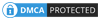#### More Stuff

Follow

Discussion Area in Excel Tutorials
Function are the most important part of excel and we need to know how to and where to use function and formula so we divided these function in the following category, it helps you to focus your function area by the working area of you.1. Count and Sum:
Count and sum these are the most useful function in the excel

2. Logical:
Logical function include IF, AND, OR function these function used for getting some logical answer

3. Cell References:
Relative,  absolute and mixed reference is the cell reference used in excel we will discuss it, Cell reference is not function but it is part of function for used

4. Date & Time:
To calculate, evaluation and date format we use date & time function

5. Text:
Used to manipulating and format for text in the cell

6. Lookup & Reference:
These functions are also very important for the data analysis VLOOKUP, HLOOKUP, MATCH, INDEX and CHOOSE function.

7. Financial:
In this area we will discuss all financial function how to used and where to used, these functions are mostly used for the financial companies

8. Statistical:
Very useful function are found in these sections if you are working in the area of research or analytical area these functions are very useful

9. Round:
The ROUND, ROUNDUP, and ROUNDDOWN function. we discuss it

10. Formula Errors:
In this area, we will discuss the error type and how to solve if the error got and what type of error is that.

##### More From Our Site:

About Us | HTML to XML Converter | Webmail Service | Nepali Unicode | Terms and Conditions | Privacy Policy | Contact Us |